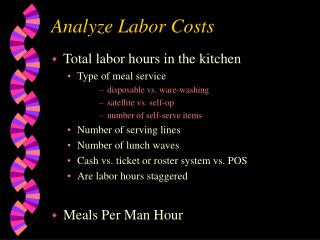DownloadDownload PresentationAnalyze Labor Costs

# Analyze Labor Costs

Télécharger la présentation## Analyze Labor Costs

- - - - - - - - - - - - - - - - - - - - - - - - - - - E N D - - - - - - - - - - - - - - - - - - - - - - - - - - -
##### Presentation Transcript

1. Analyze Labor Costs • Total labor hours in the kitchen • Type of meal service • disposable vs. ware-washing • satellite vs. self-op • number of self-serve items • Number of serving lines • Number of lunch waves • Cash vs. ticket or roster system vs. POS • Are labor hours staggered • Meals Per Man Hour

2. Meals Per Man Hour Formula 1. Calculate Average Daily Participation (ADP). ADP = total number meals served during month number of serving days in the month 2. Calculate the total daily labor hours in the kitchen. 3. Divide ADP by total daily labor hours.

3. Meals Per Man Hour Example • 5770 meals served in January 19 serving days = 304 ADP • Total daily labor hours = 17 • 304 / 17 = 17.89 = 18 MPMH • Target Range: • 20-22 MPMH with ware-washing • 22-25 MPMH all disposable

4. Meal Equivalents Formula • Total A La Carte Dollars • Meal Price = ME • Examples of a la carte income include: • a la carte sales such as snacks, bagels, and ice cream • adult lunches • student milk • adult milk

5. Determining Meal Equivalents • Determine daily a la cart income • monthly a la carte sales / number serving days ie: \$7995.80 / 19 = \$495.61 • Divide the daily a la carte \$ by meal price ie: \$495.61 / 1.50 = 330 ME • ME + ADP / daily labor hours = MPMH ie: 330 + 632 = 962 / 52.75 = 18.25 MPMH